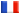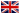# Séminaire de Cryptographie

## Jeroen Demeyer### The probability that a F_q-hypersurface is smooth

Consider the projective space P^n over a finite field F_q. A hypersurface is defined by one homogenous equation with coefficients in F_q. For d going to infinity, we show that the probability that a hypersurface of degree d is nonsingular approaches 1/\zeta_{P^n (n+1)}. This is analogous to the well-known fact that the probability that an integer is squarefree equals 1/\zeta(2) = 6/\pi^2.

This is a special case of the results in Bjorn Poonen's paper Bertini Theorems over Finite Fields'', where he computes the probability that a given variety intersects a random hypersurface. Poonen uses the full power of algebraic geometry, whereas the special case can be proven using only elementary linear algebra and properties of finite fields.